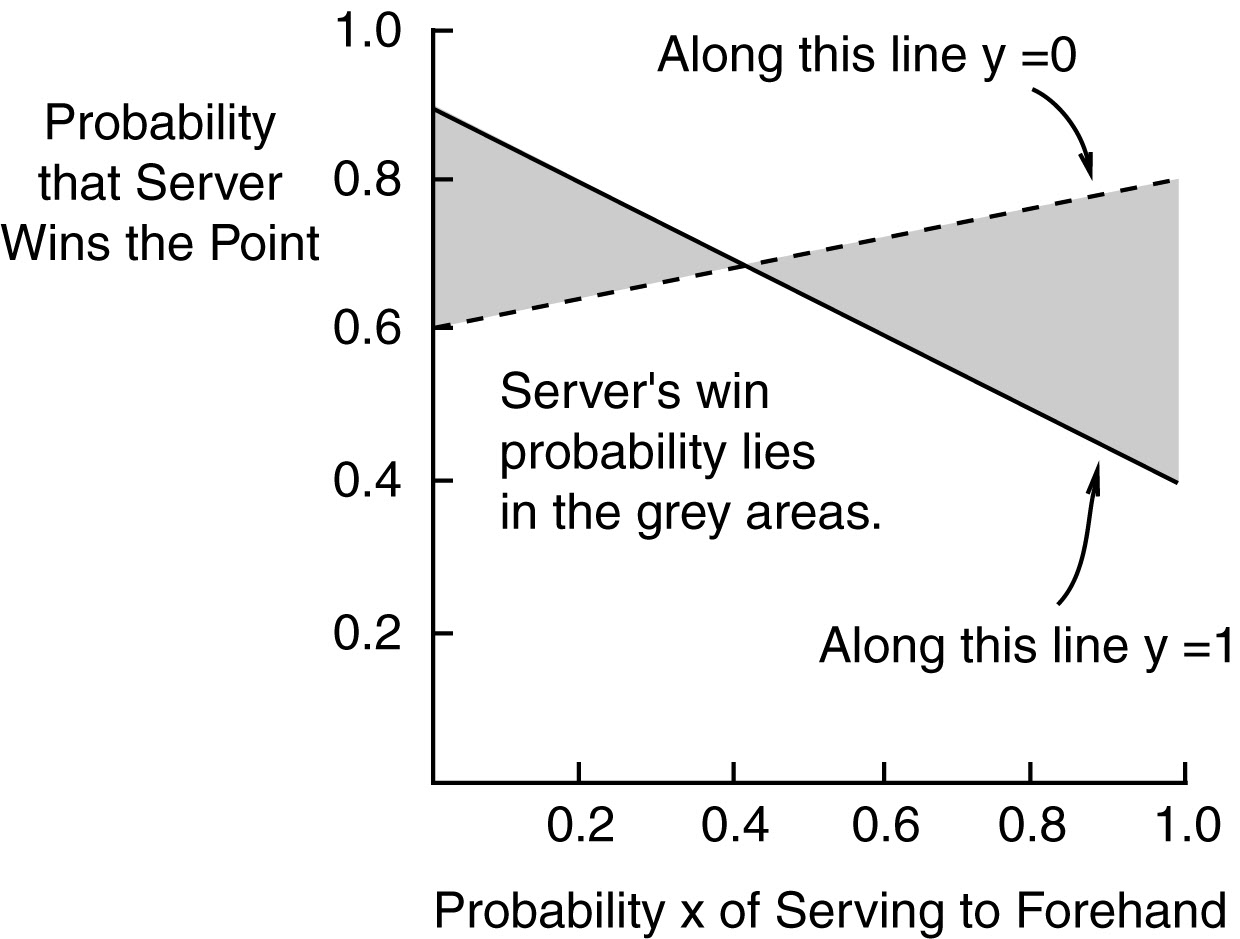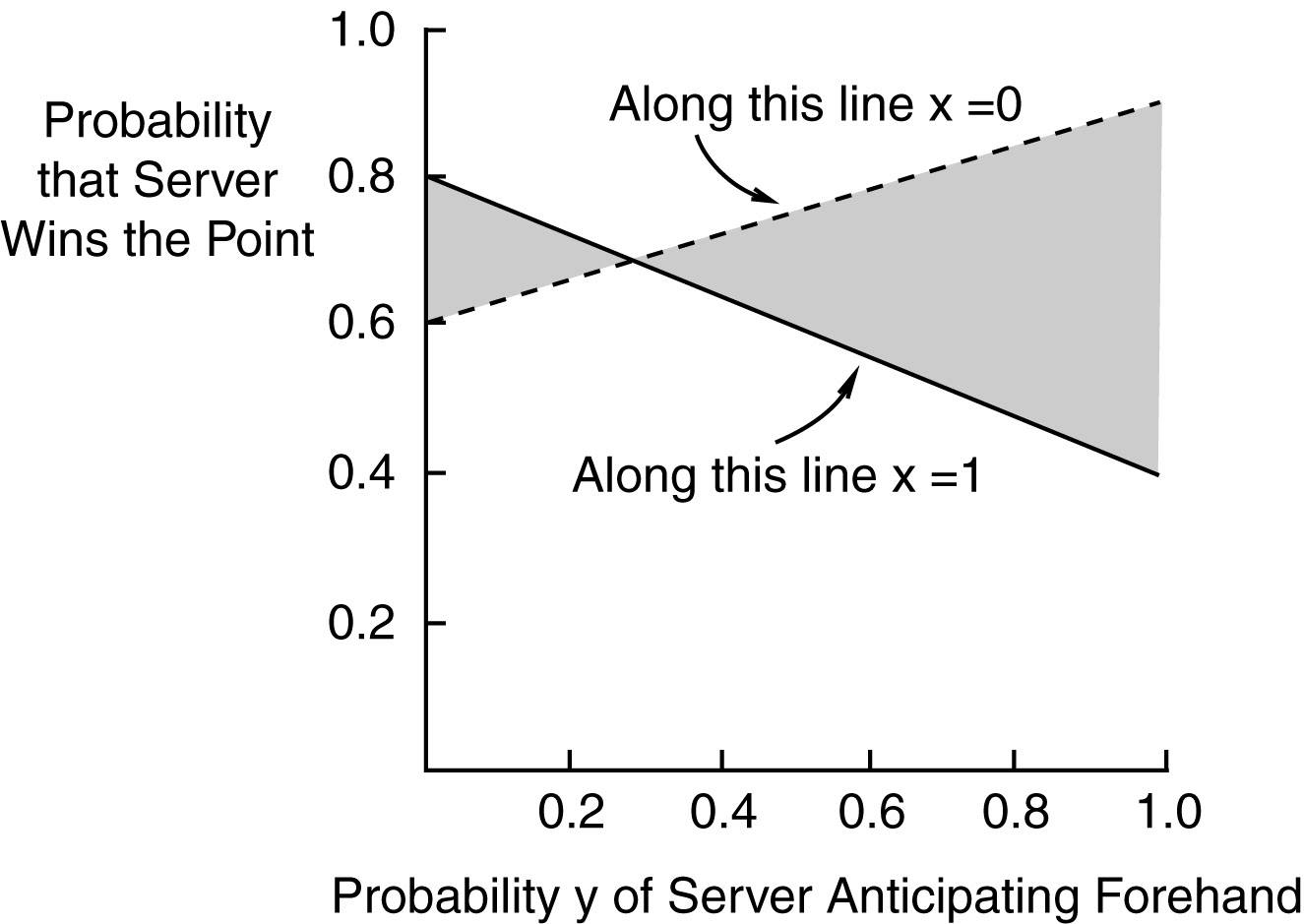Optimum Serving and Receiving Strategies in Tennis and Competetive Games in General

Many competetive situations can be simplified to or approximated as comprising two opposing teams, each having two playing options whose probability of success depends on which of two options the opponent uses. Some examples are, where to kick and defend in a soccor penalty shoot-out, whether or not to take and defend against a three point shot in basketball, whether or not to use a particular pitch in baseball, and whether or not to engage in a legally questionable practice. As a concrete example, here I will use serving and receiving in tennis.

Suppose you can serve equally well to your opponent’s forehand and backhand, and that his forehand is stronger than his backhand. In this case, you should serve exclusively to his backhand. To counter this, your opponent could anticipate (move toward that side before the ball is hit) such a serve, and then the server’s probability of winning the point will go down. To counter his opponent’s anticipating a serve to backhand, the server can occasionally serve to his opponent’s forehand, and if the opponent is leaning backhand, the server has a high probability of winning the point. Some combination of randomly serving to the forehand and backhand side must be optimum. What is the best strategy of play for each player?

To analyze, assume that there are just four possibilities, serve to either forehand or backhand, and for the receiver to anticipate a serve to forehand or anticipate a serve to backhand. The following table provides possible probabilities of the server winning the point for the four possibilities. Note that in this example, the receiver’s forehand is stronger than his backhand.

```                         Probability of Server Winning the Point
Serve to    Serve to
Forehand   Backhand

Forehand

Backhand
```

What is the best steady state strategy for each player, assuming both are rational and intelligent? That is, can the server do better than winning the 60% of the points that he would win if he served exclusively to his opponent’s backhand? (The receiver would then anticipate each serve would be to his backhand, and on each point his probability of winning would be 0.6.) If there is a better strategy for the server, what is it, and at the same time, what is the best that the receiver can do to minimize the server’s advantage? (The answer is "Yes", the server can be guaranteed to do quite a lot better than win 60% of his serves, and there is a best strategy for the receiver as well.)

Let the server serve to the forehand and backhand, randomly choosing between the two such that overall, x fraction of the serves go to forehand and 1-x of the time they go to the backhand side. Let the receiver randomly anticipate which side the serve will go to, anticipating a serve to his forehand side with a probability y, and to his backhand 1-y.

Per serve, the overall probability W that the server wins the point is the sum of the probabilities of the server winning the point for each of the four possibilities. From the four table entries above and the probabilities x and y, W = 0.4xy + 0.8x(1-y) +0.9(1-x)y + 0.6(1-x)(1-y). After combining common terms W = 0.2x + 0.3y -0.7xy + 0.6.

We can analyze this by picking different values for x and, for each of these, determining full range of probable outcomes for the full range of values for y ( 0 to 1) that the receiver may utilize. For example, if the server were to choose x = 0.2, W = 0.04 + 0.3y - 0.14y + 0.6, or W = 0.16y + 0.64. Thus, if the receiver chooses to play using a y of 0, then W = 0.64, and if he uses y = 1, then W = 0.8. For intermediate values of y, W lies between 0.64 and 0.8. Hence, if the server uses an x of 0.2, his minimum winning rate is 0.64, and his maximum winning rate is 0.8. The first graph shows in grey for each value of x, the range of potential winning probabilities W possible.

The server should assume that the receiver is intelligent and uses the best possible strategy to minimize what the server can win for each value of x. Thus the server should use the value of x for which his minimum winning percentage has the maximum value. From the graph this can be seen to occur at an x a little above 0.4 (actually is 0.428, and W = 0.686).What about the receiver’s strategy? Clearly, he wants to minimize W. The same type of analysis applies, and the second graph shows the range of W possible for each value of y chosen by the receiver. To find the best strategy for the receiver, consider the second graph, which shows the range of W possible for each value of y chosen by the receiver. In this case, the range of W varies depending on what value x the server chooses.The receiver should use a value of y for which the server’s maximum winning probability W is a minimum. From the graph, this can be seen to be when y is a little less than 0.3 (0.286 and W = 0.686). At this point, which it must, for at the stable point, the strategies used by both players should predict the same probability that the server wins the point.

It is interesting to note that at the extremes for the server, x = 0, and x = 1.0, variation of the receiver’s response y produces the largest (local) changes in W, and similarly, at the extremes of the receiver’s behavior, y = 0 and y = 1, variation of the server’s response x produces the largest (local) change in W. The long term stable point in the strategies of both players is for server to use an x of 0.428 and for the receiver to use a y of 0.286. It is also interesting to note that if the server uses his optimum value for x, it doesn’t matter at all what the receiver anticipates. Similarly, if the receiver anticipates his optimum value for y, it doesn’t matter what value for x that the server uses. However, if a player continuously uses a nonoptimum x or y, his opponent can shift his strategy and thereby do better than he would at the optimum point. Perhaps analyses like this will generate a market for tennis rackets with built in random number generators?

From the server’s point of view W of 0.686 is quite a bit better than 0.6, and from the receiver’s point of view is not nearly as bad as it could be. The difference between winning 60% and 70% makes a big difference overall in a normal tennis match, see http://pages.jh.edu/~rschlei1/Random_stuff/tennis.html. Thus there is a substantial reward for being smart enough not to serve exclusively to this opponent’s backhand.

For a general case, if we replace the values in the first table with symbols, and solve,

```
Probability of Server Winning the Point
Serve to     Serve to
Forehand   Backhand